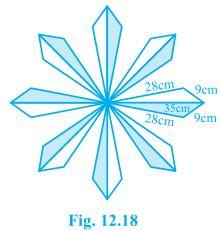# A floral design on a floor is made up of 16 tiles which are triangular, the sides of the triangle being 9 cm, 28 cm and 35 cm (see Fig) . Find the cost of polishing the tiles at the rate of 50p per cm2The area for each triangle will be:

Now, for each ar(△)can be calculated by Heron’s Formula, where

a = 9 cm

b = 28 cm

c = 35 cm

Semi Perimeter (s) = a+b+c/2

s = 9+28+35/2

s=36

ar(△) = √s(s-a)(s-b)(s-c)

= √36(36-9)(36-28)(36-35)

= √36×(27)×(8)×(1)

= 36√6 cm2

As there are 16 tiles, so total area = 16 × 36√6

= 1410.906 cm2

As 1 cm= 50 p = ₹ 0.5

1410.906 cm2 = ₹ 0.5 ×1410.906

= ₹ 705.45

The total polishing cost of the tiles = Rs. 705.6